# 机器学习-吴恩达：学习笔记及总结（6）

## Coursera | Machine Learning | Andrew Ng

Thistledown    March 22, 2020

## 16 Evaluating a Learning Algorithm

#### 16.1 Deciding What to Try Next

• Debugging a learning algorithm:

• Suppose you have implemented regularized linear regression to predict housing prices.

• However, when you test your hypothesis on a new set of houses, you find that it makes unacceptably large errors in its predictions. What should you try next?

• Get more training examples
• Try smaller sets of features
• Try adding polynomial features $(x_1^2,\ x_2^2,\ x_1x_2,\ \text{etc.})$
• Try decreasing/increasing $\lambda$
• Machine learning diagnostic:

• Diagnostic: A test that you can run to gain insight what is/isn’t working with a learning algorithm, and gain guidance as to how best to improve its performance.
• Diagnostic can take time to implement, but doing so can be a very good use of your time.

#### 16.2 Evaluating a Hypothesis

• Once we have done some troubles shooting for errors in our predictions by:

• Getting more training examples
• Trying smaller sets of features
• Trying polynomial features
• Increasing or decreasing $\lambda$

We can move on to evaluate our new hypothesis

• A hypothesis may have a low error for the training examples but still be inaccurate (because of overfitting). Thus, to evaluate a hypothesis, given a dataset of training examples, we can split up the data into two sets: a training set and a test set. Typically, the training set consists of 70% of your data and the test set is the remaining 30%.

• The new procedure using these two sets is then:

• Learn $\Theta$ and minimize $J_{\text{train}}(\Theta)$ using the training set
• Compute the test set error $J_{\text{test}}(\Theta)$
• The test set error

• For linear regression:

• For classification - Misclassification error (aka 0/1 misclassification error):

This gives us a binary 0 or 1 error result based on a misclassification.

• The average test error for the test set is:

This gives us the proportion of the test data that was misclassified.

#### 16.3 Model Selection and Train/Validation/Test Sets

• Just because a learning algorithm fits a training set well, that does not mean it is a good hypothesis. It could over fit and as a result your predictions on the test set would be poor.

• The error of your hypothesis as measured on the data set with which you trained the parameters will be lower than the error on any other data set.

• Given many models with different polynomial degrees, we can use a systematic approach to identify the “best” function. In order to choose the model of your hypothesis, you can test each degree of polynomial and look at the error result.

• One way to break down our dataset into the three sets is:

• Training set: 60%
• Cross validation set: 20%
• Test set: 20%
• Train/validation/test error

• Training error:
• Cross validation error:
• Test error:
• We can now calculate three separate error values for the three different sets using the following method:

• Optimize the parameters in $\Theta$ using the training set for each polynomial degree.
• Find the polynomial degree $d$ with the least error using the cross validation set.
• Estimate the generalization error using the test set with $J_{\text{test}}\left(\Theta^{(d)}\right)$, $d$ = theta from polynomial with lower error.
• This way, the degree of the polynomial $d$ has not been trained using the test set.

## 17 Bias vs. Variance

#### 17.1 Diagnosing Bias vs. Variance

• Bias/variance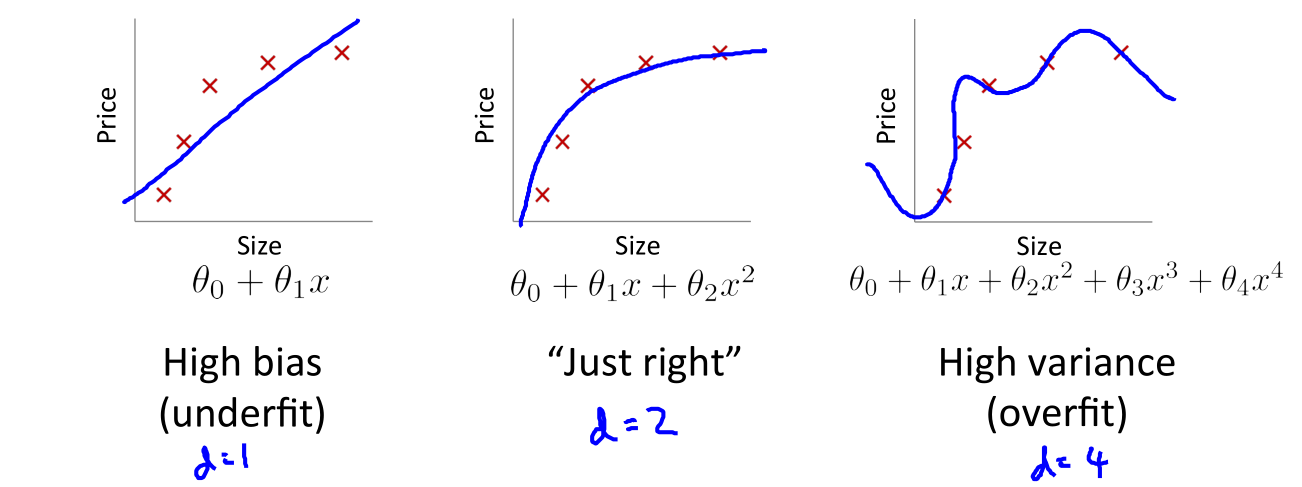• In this section we examine the relationship between the degree of the polynomial $d$ and the underfitting or overfitting of our hypothesis.

• We need to distinguish whether bias or variance is the problem contributing to bad predictions.
• High bias is underfitting and hign variance is overfitting. Ideally, we need to find a golden mean between these two.
• The training error will tend to decrease as we increase the degree $d$ of the polynomial.

• At the same time, the cross validation error will tend to decrease as we increase $d$ up to a point, and then it will increase as $d$ is increased, forming a convex curve.

• High bias (underfitting): both $J_{\text{train}}(\Theta)$ and $J_{\text{CV}}(\Theta)$ will be high. Also, $J_{\text{CV}}(\Theta) \approx J_{\text{train}}(\Theta)$.
• High variance (overfitting): $J_{\text{train}}(\Theta)$ will be low and $J_{\text{CV}}(\Theta)$ will be much greater than $J_{\text{train}}(\Theta)$.

This is summarized in the figure below: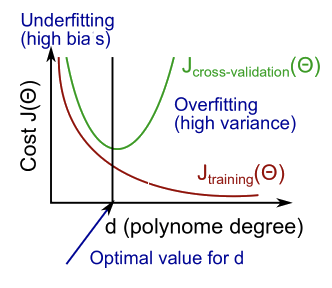#### 17.2 Regularization and Bias/Variance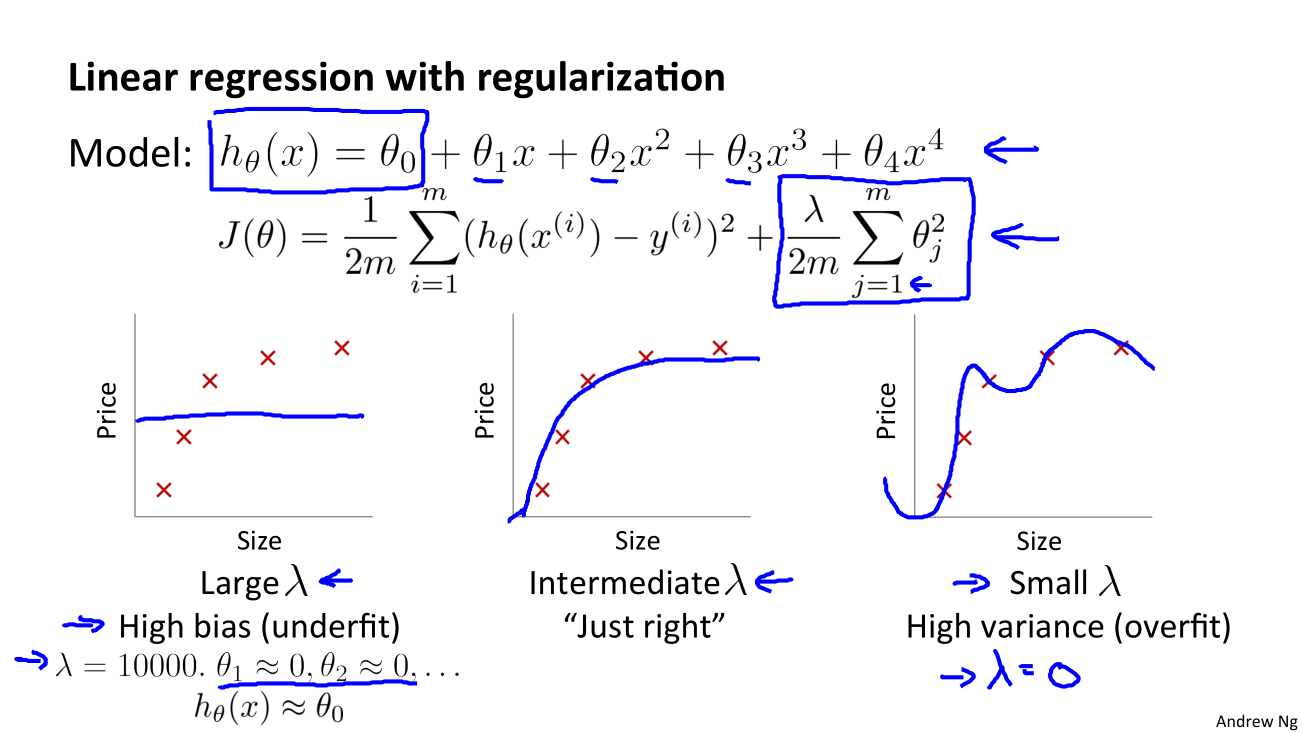In the figure above (The regularization term should be $\frac{\lambda}{2m} \sum_{j=1}^n\theta_j^2$), we see that as $\lambda$ increases, our fit becomes more rigid. On the other hand, as $\lambda$ approaches 0, we tend to overfit the data. So how do we choose our parameter $\lambda$ to get it “just right”? In order to choose the model and the regularization term $\lambda$, we need to:

1. Create a list of lambdas (e.g. $\lambda \in \lbrace 0,\,0.01,\,0.02,\,0.04,\,0.08,\,\dots,10.24 \rbrace$).
2. Create a set of models with different degrees or any other variants.
3. Iterate through the $\lambda$s and for each $\lambda$ go through all the models to learn some $\Theta$.
4. Compute the cross validation error using the learned $\Theta$ (computed with $\lambda$) on the $J_{\text{CV}}(\Theta)$ without regularization or $\lambda = 0$.
5. Select the best combo that produces the lowest error on the cross validation set.
6. Using the best combo $\Theta$ and $\lambda$, apply it on $J_{\text{test}}(\Theta)$ to see if it has a good generalization of the problem.

#### 17.3 Learning Curves

• Training an algorithm on a very few number of data points (such as 1, 2 or 3) will easily have 0 errors because we can always find a quadratic curve that touches exactly those number of points. Hence:

• As the training set gets larger, the error for a quadratic function increases.
• The error value will plateau out after a certain $m$, or training set size.
• Experiencing high bias:

• Low training set size: causes $J_{\text{train}}(\Theta)$ to be low and $J_{\text{CV}}(\Theta)$ to be high.
• Large training set size: causes both $J_{\text{train}}(\Theta)$ and $J_{\text{CV}}(\Theta)$ to be high with $J_{\text{train}}(\Theta)\approx J_{\text{CV}}(\Theta)$.
• If a learning algorithm is suffering from high bias, getting more training data will not (by itself) help much.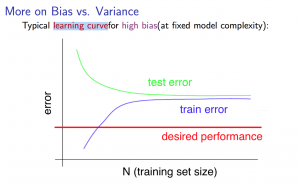• Experiencing high variance:

• Low training set size: $J_{\text{train}}(\Theta)$ will be low and $J_{\text{CV}}(\Theta)$ will be high.
• Large training set size: $J_{\text{train}}(\Theta)$ increases with training set size and $J_{\text{CV}}(\Theta)$ continues to decrease without leveling off. Also, $J_{\text{train}}(\Theta)< J_{\text{CV}}(\Theta)$ but the difference between them remains significant.
• If a learning algorithm is suffering from high variance, getting more training data is likely to help.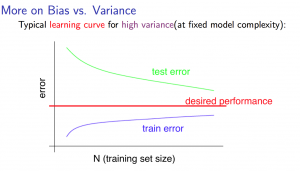#### 17.4 Deciding What to Do Next (Revisited)

• Our decision process can be broken down as follows:
• Getting more training examples: Fixes high variance
• Trying smaller sets of features: Fixes high variance
• Adding features: Fixes high bias
• Adding polynomial features: Fixes high bias
• Decreasing $\lambda$: Fixes high bias
• Increasing $\lambda$: Fixes high variance
• Diagnosing Neural Networks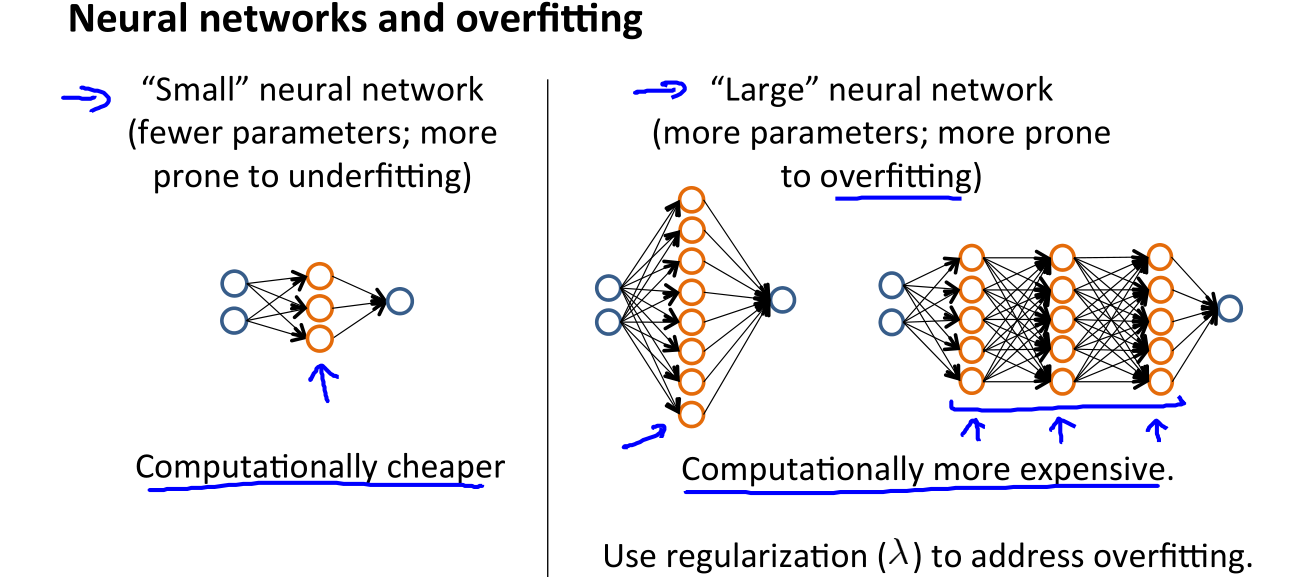• A neural network with fewer parameters is prone to underfitting. It is also computationally cheaper.
• A large neural network with more parameters is prone to overfitting. It is also computationally expensive. In this case you can use regularization (increase $\lambda$) to address the overfitting.
• Using a single hidden layer is a good starting default. You can train you neural network on a number of hidden layers using your cross validation set. You can then select the one that performs best.
• Model complexity effects
• Lower-order polynomial (low model complexity) have high bias and low variance. In this case, the model fits poorly consistently.
• Higher-order polynomial (high model complexity) fit the training data extremely well and the test data extremely poorly. These have low bias on the training data, but very high variance.
• In reality, we would want to choose a model somewhere in between, that can generalize well but also fits the data reasonably well.

## 18 Building a Spam Classifier

#### 18.1 Prioritizing What to Work On

• Supervised learning.
• $x = \text{features of email}$. Choose 100 words indicative of spam/not spam
• Note: In practice, take most frequently occurring $n$ words (10,000 to 50,000) in training set, rather that manually pick 100 words.
• $y =1 \text{ (spam), or } 0 \text{ (not spam)}$
• Building a spam classifier: How to spend your time to make it have low error?
• Collect lots of data (for example “honeypot” project but doesn’t always work).
• Develop sophisticated features based on email routing information (from email header)
• Develop sophisticated features for message body, e.g. should “discount” and “discounts” be treated as the same word? How about “deal” and “Dealer”? Features about punctuation?
• Develop sophisticated algorithm to detect misspellings (e.g. m0rtage, med1cine, w4tches).

#### 18.2 Error Analysis

• Recommended approach
• Start with a simple algorithm that you can implement quickly. Implement it and test it on your cross-validation data.
• Plot learning curves to decide if more data, more features, etc. are likely to help.
• Error analysis: Manually examine the examples (in cross validation set) that your algorithm made errors on. See if you spot any systematic trend in what type of examples it is making errors on.
• Error analysis
• The importance of numerical evaluation

## 19 Handling Skewed Data

#### 19.1 Error Metrics for Skewed Classes

• Cancer classification example

• Train logistic regression model $h_{\theta}(x)$. $y = 1 \text{ (if cancer)}$, $y=0 \text{ (otherwise)}$
• Find that you got 1% error on test set (99% correct diagnose)
• Precision/Recall

• $y = 1$ in presence of rare class that we want to detect

• Precision: of all where we predicted $y = 1$, what fraction actually has cancer? $\text{Precision} = \frac{\text{True positives}}{\text{# predicted as positive}} = \frac{\text{True positives}}{\text{True positives + False positives}}$

• Recall: of all patients that actually have cancer, what fraction did we correctly detect as having cancer? $\text{Recall} = \frac{\text{True positives}}{\text{# actual positives}} = \frac{\text{True positives}}{\text{True positives + False negatives}}$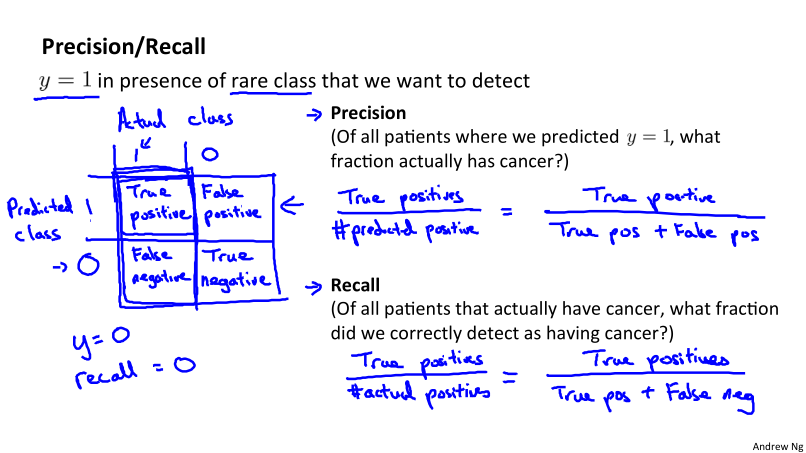#### 19.2 Trading off Precision and Recall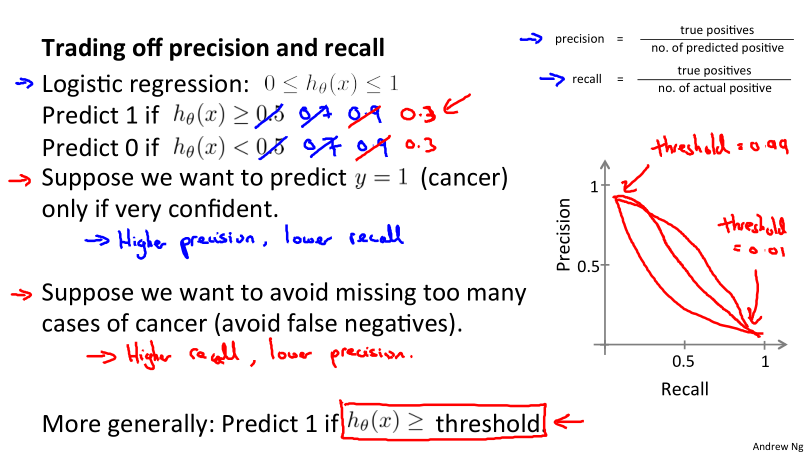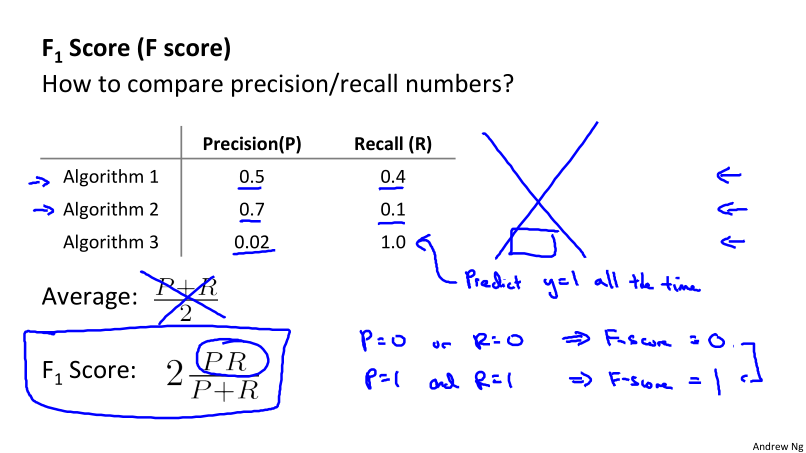## 20 Using Large Data Sets

#### 20.1 Data For Machine Learning

• Designing a high accuracy learning system
• E.g. Classify between confusable words {to, two, too}, {then than}.
• Algorithms:
• Perceptron (logistic regression)
• Winnow
• Memory-based
• Naïve Bayes
• Large data rationale
• Assume feature $x \in \Bbb{R}^{n + 1}$ has sufficient information to predict $y$ accurately.
• Use a learning algorithm with many parameters (e.g. logistic regression/linear regression with many features; neural network with many hidden units) → low bias.
• Use a very large training set (unlikely to overfit) - low variance.

## Ex5: Regularized Linear Regression and Bias v.s. Variance👨‍💻

See this exercise on Coursera-MachineLearning-Python/ex5/ex5.ipynb

#### Instruction

1. Regularization Linear Regression: In the first half of the exercise, you will implement regularized linear regression to predict the amount of water flowing out of a dam using the change of water level in a reservoir. In the next half, you will go through some diagnostics of debugging learning algorithms and examine the effects of bias v.s. variance.
• Visualizing the dataset
• Regularized linear regression cost function
• Selecting $\lambda$ using a cross validation set